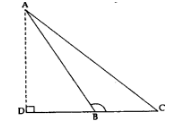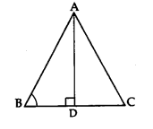# CBSE Class 10 Maths Notes & Important Questions Chapter 6 Triangles## CBSE Class 10 Maths Notes Chapter 6 Triangles

Introduction: In geometry, a triangle is a closed shape made up of three straight lines called sides. A triangle has three angles and three vertices. The sum of the angles in a triangle is always 180 degrees. There are several types of triangles based on the length of sides and measure of angles.

Types of Triangles:

1. Scalene Triangle: A scalene triangle is a triangle in which all three sides are of different lengths.
2. Isosceles Triangle: An isosceles triangle is a triangle in which two sides are of the same length.
3. Equilateral Triangle: An equilateral triangle is a triangle in which all three sides are of the same length.
4. Right Triangle: A right triangle is a triangle in which one angle is a right angle (90 degrees).

Properties of Triangles:

1. The sum of the lengths of any two sides of a triangle is greater than the length of the third side.
2. The measure of each angle in a triangle is less than 180 degrees.
3. The sum of the measures of the angles in a triangle is always 180 degrees.
4. In an isosceles triangle, the angles opposite to the equal sides are equal.
5. In a right triangle, the sum of the squares of the lengths of the two shorter sides is equal to the square of the length of the longest side (Pythagoras theorem).
6. The median of a triangle is a line segment joining a vertex to the midpoint of the opposite side.
7. The altitude of a triangle is a perpendicular line segment from a vertex to the opposite side or to an extension of the opposite side.

Important Formulas:

1. Area of a Triangle = 1/2 x base x height
2. Heron’s formula: The area of a triangle with sides a, b and c is given by:

Area = √s(s – a)(s – b)(s – c)

where s = (a+b+c)/2 is the semi-perimeter of the triangle.

1. Pythagoras theorem: In a right triangle with sides a, b and c (where c is the hypotenuse), the square of the length of the hypotenuse is equal to the sum of the squares of the lengths of the other two sides.

c^2 = a^2 + b^2

1. Sum of the angles of a triangle: The sum of the three angles of a triangle is 180 degrees.

Applications of Triangles:

1. Triangles are used in architecture and construction to calculate the height, length, and width of structures.
2. In trigonometry, triangles are used to find the relationship between angles and sides.
3. In navigation, triangles are used to determine distances between two points.
4. Triangles are used in art and design to create a sense of balance and symmetry.

## CBSE Class 10 Maths Important Questions Chapter 6 Triangles

Important Questions:

1. What is the sum of the angles in a triangle?
2. What is an isosceles triangle?
3. What is the Pythagoras theorem?
4. What is the area of a triangle?
5. What is Heron’s formula?
6. What is the altitude of a triangle?
7. What is the median of a triangle?
8. In a right triangle, which side is the hypotenuse?
9. What is the sum of the angles in a quadrilateral?
10. What is the relationship between the angles and sides of a triangle in trigonometry?

## CBSE Class 10 Maths Important Questions Answers Chapter 6 Triangles

1. In ΔABC, AB = AC and AD is an altitude. Prove that AD bisects BC.

Solution: Given, AB = AC and AD is an altitude of ΔABC. To prove: AD bisects BC Proof: Since, AB = AC, therefore, ∠ABC = ∠ACB …(1) [Angle opposite to equal sides are equal] In ΔABD and ΔACD, AB = AC [Given] AD = AD [Common] ∠ABD = ∠ACD = 90° [Given] Therefore, by AA similarity, ΔABD ≅ ΔACD Hence, BD = CD …(2) [Corresponding parts of congruent triangles are equal] From equation (1) and (2), we have: BD = CD and ∠ABC = ∠ACB Therefore, by Converse of Basic Proportionality Theorem, AD bisects BC.

1. In ΔABC, ∠A = 90° and AD is the median from A. Prove that AB² + AC² = 2AD².

Solution: Given, in ΔABC, ∠A = 90° and AD is the median from A. To prove: AB² + AC² = 2AD² Proof: In ΔABD, ∠A = 90° Therefore, by Pythagoras theorem, we have: AB² = AD² + BD²…(1) Similarly, in ΔACD, ∠A = 90° Therefore, by Pythagoras theorem, we have: AC² = AD² + CD²…(2) Adding equation (1) and (2), we get: AB² + AC² = 2AD² + BD² + CD² …(3) Now, since D is the midpoint of BC, we have: BD = CD Therefore, BD² = CD² Substituting this value in equation (3), we get: AB² + AC² = 2AD² + 2BD² = 2(AD² + BD²) = 2AD² Hence, proved.

1. In ΔABC, D is a point on BC such that BD/DC = AB/AC. Prove that AD is the bisector of ∠A.

Solution: Given, in ΔABC, D is a point on BC such that BD/DC = AB/AC. To prove: AD is the bisector of ∠A. Proof: Let us assume BD = x and DC = y Therefore, we have: BD/DC = AB/AC x/y = AB/AC ACx = AB(y) [Cross multiplying] Dividing throughout by (x + y), we get: ACx/(x + y) = AB(y)/(x + y) AC/AD = AB/BD [AD = x + y] Therefore, by Converse of Basic Proportionality Theorem, we have: AD bisects ∠A. Hence, proved.

1. In ΔABC, DE || BC, where D is on AB and E is on AC. Prove that AD/DB = AE/EC.

Solution: Given, in ΔABC, DE || BC, where D is on AB and E is on AC. To prove: AD/DB = AE/EC Proof: Since, DE || BC, therefore, by Basic Proportionality Theorem, we have: AD/DB = AE/EC Hence, proved.

## CBSE Class 10 Maths Important Questions Answers MCQs Chapter 6 Triangles

In a right triangle ABC with hypotenuse AC, if AB = 6 and BC = 8, then what is the value of AC?
a) 10
b) 12
c) 14
d) 15

In ΔABC, AD is an altitude. If AB = 12 and BD = 8, what is the value of CD?
a) 10
b) 6
c) 4
d) 2

In a triangle ABC, if AB = 5, BC = 12 and AC = 13, then what is the measure of ∠BAC?
a) 30°
b) 45°
c) 60°
d) 90°

If two angles of a triangle measure 40° and 70°, what is the measure of the third angle?
a) 40°
b) 50°
c) 60°
d) 70°

If two sides of a triangle are 6 cm and 8 cm, what is the possible range of the length of the third side?
a) 1 cm < x < 14 cm
b) 2 cm < x < 14 cm
c) 3 cm < x < 14 cm
d) 4 cm < x < 14 cm
Answer: c) 3 cm < x < 14 cm

## CBSE Class 10 Maths Notes Chapter 6 Triangles

SIMILAR FIGURES

• Two figures having the same shape but not necessary the same size are called similar figures.
• All congruent figures are similar but all similar figures are not congruent.

SIMILAR POLYGONS
Two polygons are said to be similar to each other, if:
(i) their corresponding angles are equal, and
(ii) the lengths of their corresponding sides are proportional

Example:
Any two line segments are similar since length are proportional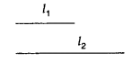Any two circles are similar since radii are proportionalAny two squares are similar since corresponding angles are equal and lengths are proportional.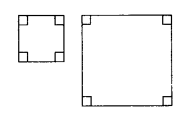Note:
Similar figures are congruent if there is one to one correspondence between the figures.
∴ From above we deduce:

Any two triangles are similar, if their(i) Corresponding angles are equal
∠A = ∠P
∠B = ∠Q
∠C = ∠R

(ii) Corresponding sides are proportional
ABPQ=ACPR=BCQR

THALES THEOREM OR BASIC PROPORTIONALITY THEORY

Theorem 1:
State and prove Thales’ Theorem.
Statement:
If a line is drawn parallel to one side of a triangle to intersect the other two sides in distinct points, the other two sides are divided in the same ratio.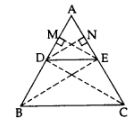Given: In ∆ABC, DE || BC.
Const.: Draw EM ⊥ AD and DN ⊥ AE. Join B to E and C to D.
∵ DE || BC …[Given
∴ ar(∆BDE) = ar(∆CDE)
…[∵ As on the same base and between the same parallel sides are equal in area
From (i), (ii) and (iii),

CRITERION FOR SIMILARITY OF TRIANGLES

Two triangles are similar if either of the following three criterion’s are satisfied:

• AAA similarity Criterion. If two triangles are equiangular, then they are similar.
• Corollary(AA similarity). If two angles of one triangle are respectively equal to two angles of another triangle, then the two triangles are similar.
• SSS Similarity Criterion. If the corresponding sides of two triangles are proportional, then they are similar.
• SAS Similarity Criterion. If in two triangles, one pair of corresponding sides are proportional and the included angles are equal, then the two triangles are similar.

Results in Similar Triangles based on Similarity Criterion:

1. Ratio of corresponding sides = Ratio of corresponding perimeters
2. Ratio of corresponding sides = Ratio of corresponding medians
3. Ratio of corresponding sides = Ratio of corresponding altitudes
4. Ratio of corresponding sides = Ratio of corresponding angle bisector segments.

AREA OF SIMILAR TRIANGLES

Theorem 2.
The ratio of the areas of two similar triangles is equal to the square of the ratio of their corresponding sides.
Given: ∆ABC ~ ∆DEF
To prove: ar(ΔABC)ar(ΔDEF)=AB2DE2=BC2EF2=AC2DF2
Const.: Draw AM ⊥ BC and DN ⊥ EF.
Proof: In ∆ABC and ∆DEFar(ΔABC)ar(ΔDEF)=12×BC×AM12×EF×DN=BCEF.AMDN …(i) ……[Area of ∆ = 12 x base x corresponding altitude
∵ ∆ABC ~ ∆DEF
∴ ABDE=BCEF …..(ii) …[Sides are proportional
∠B = ∠E ……..[∵ ∆ABC ~ ∆DEF
∠M = ∠N …..[each 90°
∴ ∆ABM ~ ∆DEN …………[AA similarity
∴ ABDE=AMDN …..(iii) …[Sides are proportional
From (ii) and (iii), we have: BCEF=AMDN …(iv)
From (i) and (iv), we have: ar(ΔABC)ar(ΔDEF)=BCEF.BCEF=BC2EF2
Similarly, we can prove that
ar(ΔABC)ar(ΔDEF)=AB2DE2=AC2DF2
∴ar(ΔABC)ar(ΔDEF)=AB2DE2=BC2EF2=AC2DF2

Results based on Area Theorem:

1. Ratio of areas of two similar triangles = Ratio of squares of corresponding altitudes
2. Ratio of areas of two similar triangles = Ratio of squares of corresponding medians
3. Ratio of areas of two similar triangles = Ratio of squares of corresponding angle bisector segments.

Note:
If the areas of two similar triangles are equal, the triangles are congruent.

PYTHAGORAS THEOREM

Theorem 3:
State and prove Pythagoras’ Theorem.
Statement:
Prove that, in a right triangle, the square of the hypotenuse is equal to the sum of the squares of the other two sides.
Given: ∆ABC is a right triangle right-angled at B.
To prove: AB² + BC² = AC²
Const.: Draw BD ⊥ AC
Proof: In ∆s ABC and ADB,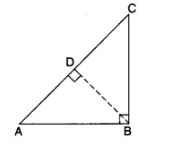∠A = ∠A …[common
∴ ∆ABC ~ ∆ADB …[AA Similarity
Now in ∆ABC and ∆BDC
∠C = ∠C …..[common]
∠ABC = ∠BDC ….[each 90°]
∴ ∆ABC ~ ∆BDC …..[AA similarity]
∴ BCDC=ACBC ……..[sides are proportional]
BC² = AC.DC …(ii)
On adding (i) and (ii), we get
AB² + BC² = ACAD + AC.DC
⇒ AB² + BC² = AC.(AD + DC)
AB² + BC² = AC.AC
∴AB² + BC² = AC²

CONVERSE OF PYTHAGORAS THEOREM

Theorem 4:
State and prove the converse of Pythagoras’ Theorem.
Statement:
Prove that, in a triangle, if square of one side is equal to the sum of the squares of the other two sides, then the angle opposite the first side is a right angle.Given: In ∆ABC, AB² + BC² = AC²
To prove: ∠ABC = 90°
Const.: Draw a right angled ∆DEF in which DE = AB and EF = BC
Proof: In ∆ABC,
AB² + BC² = AC² …(i) [given]
In rt. ∆DEF
DE² + EF² = DF² …[by pythagoras theorem]
AB² + BC² = DF² …..(ii) …[DE = AB, EF = BC]
From (i) and (ii), we get
AC² = DF²
⇒ AC = DF
Now, DE = AB …[by cont]
EF = BC …[by cont]
DF = AC …….[proved above]
∴ ∆DEF ≅ ∆ABC ……[sss congruence]
∴ ∠DEF = ∠ABC …..[CPCT]
∠DEF = 90° …[by cont]
∴ ∠ABC = 90°

Results based on Pythagoras’ Theorem:
(i) Result on obtuse Triangles.
If ∆ABC is an obtuse angled triangle, obtuse angled at B,